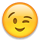## Forum Stats

• 3,752,633 Users
• 2,250,529 Discussions

Discussions

# Multi-Rows from DUAL

• Yes, there is. The difference would be that Oracle
would now have 1 row to put into the model initially
and it would have to administer which rows where
original and which ones are new. The query as is
starts blank, generates 10 cells, and converts them
back as rows. A little less work than using the
RETURN UPDATED ROWS clause.
Well, If I fully understand what you mean - I can't agree.
Cause when you use e.g. WHERE 1=2 in the model clause it would return not only inserted values, but also updated - so the process would be quite the same - it also have to recognize what values were updated and inserted, and what values - were not touched.
```SQL> with t as (select 1 num from dual union all
2             select 2 from dual union all
3             select 3 from dual union all
4             select 4 from dual)
5             --
6             select * from t
7  --            where 1=2
8              model
9               return updated rows
10               dimension by (num rn)
11               measures(num)
12                rules(num=0,
13                      num=0,
14                      num=-1,
15                      num=-1)
16  /

RN        NUM
---------- ----------
1          0
3          0
6         -1
5         -1

SQL>
SQL> with t as (select 1 num from dual union all
2             select 2 from dual union all
3             select 3 from dual union all
4             select 4 from dual)
5             --
6             select * from t
7              where 1=2
8              model
9  --             return updated rows
10               dimension by (num rn)
11               measures(num)
12                rules(num=0,
13                      num=0,
14                      num=-1,
15                      num=-1)
16  /

RN        NUM
---------- ----------
6         -1
5         -1
3          0
1          0

SQL> ```
• SQL> ed
Wrote file afiedt.buf

1 SELECT rownum
2 FROM Dual
3* CONNECT BY rownum <= 10
L> /

ROWNUM
------
1
showing only 1 not 1 to 10
That's because you are using an earlier version of Oracle.

Try:

SELECT rn FROM (SELECT rownum rn FROM DUAL CONNECT BY ROWNUM <= 10);• Ooops, realized that I'm wrong!
They are all four rows inserted - you are right, Rob.

If we use symbolic reference - we can easily see the difference:
```SQL> with t as (select 1 num from dual union all
2             select 2 from dual union all
3             select 3 from dual union all
4             select 4 from dual)
5             --
6             select * from t
7  --            where 1=2
8              model
9               return updated rows
10               dimension by (num rn)
11               measures(num)
12                rules(num[rn=1]=0,
13                      num[rn=3]=0,
14                      num=-1,
15                      num=-1)
16  /

RN        NUM
---------- ----------
1          0
3          0
6         -1
5         -1

SQL>
SQL> with t as (select 1 num from dual union all
2             select 2 from dual union all
3             select 3 from dual union all
4             select 4 from dual)
5             --
6             select * from t
7              where 1=2
8              model
9  --             return updated rows
10               dimension by (num rn)
11               measures(num)
12                rules(num[rn=1]=0,
13                      num[rn=3]=0,
14                      num=-1,
15                      num=-1)
16  /

RN        NUM
---------- ----------
6         -1
5         -1

SQL> ```
• The reason I used that condition is explained in the section labelled "WHERE 1=2" at SQL Snippets: Integer Series Generators - MODEL Method.

Volder's right about RETURN UPDATED ROWS though. It would produce the same results as using WHERE 1=2.

--
Joe Fuda
SQL Snippets
• The best solution is CONNECT BY as other said,

But if you forget it , one simple trick is just select rownum from dba_tables where rownum<10000.

Regards
Helio Dias
http://heliodias.com
• Volder's right about RETURN UPDATED ROWS though. It
would produce the same results as using WHERE 1=2.
No, Joe, I wasn't right. And I gave an example in my post previous to your post• Sorry Volder, I don't follow. I was saying that this
```select integer_value
from   dual
-- where 1=2
model
RETURN UPDATED ROWS
dimension by ( 0 as key )
measures     ( 0 as integer_value )
rules upsert ( integer_value[ for key from 1 TO 3 increment 1 ] = cv(key) )
;
INTEGER_VALUE
-------------
1
2
3```
gives the same results as this
```select integer_value
from   dual
where 1=2
model
--  RETURN UPDATED ROWS
dimension by ( 0 as key )
measures     ( 0 as integer_value )
rules upsert ( integer_value[ for key from 1 TO 3 increment 1 ] = cv(key) )
;
INTEGER_VALUE
-------------
1
2
3```
As an aside, for many cases we don't need either clause. The following version works fine for queries with hardcoded limits that always return one or more rows.
```select integer_value
from   dual
model
dimension by ( 1 as key )            -- use "1" here instead of "0"
measures     ( 1 as integer_value )  -- use "1" here instead of "0"
rules upsert ( integer_value[ for key from 1 TO 3 increment 1 ] = cv(key) )
;
INTEGER_VALUE
-------------
1
2
3```
Personally I prefer the WHERE 1=2 approach because it seems cleaner and safer to start with an empty set right before the MODEL rules are applied. That's just me though.

--
Joe Fuda
SQL Snippets
• SELECT Row_Number() over(order by 1) FROM DUAL CONNECT BY ROWNUM <11;
• user12919849 wrote:
SELECT Row_Number() over(order by 1) FROM DUAL CONNECT BY ROWNUM <11;
Ok, so you've dragged up an old old thread for what reason?

There's absolutely no benefit in using the analytical function row_number() to get row numbers out of that query when rownum itself will do the job. Using that function will only serve to decrease performance.
This discussion has been closed.